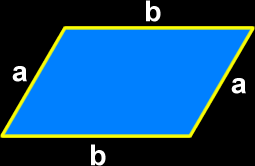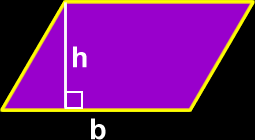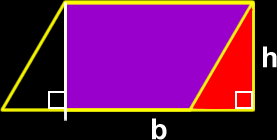# The Properties of a Parallelogram

Definitions and formulas for the perimeter of a parallelogram, the area of a parallelogram, properties of the sides and angles of a parallelogram
Just scroll down or click on what you want and I'll scroll down for you!

 perimeter of a parallelogram area of a parallelogram properties of the sidesand angles of a parallelogram other properties of theangles of a parallelogram

 The perimeter of a parallelogram:To find the perimeter of a parallelogram, just add up all the lengths of the sides:Perimeter = a + b + a + b        = 2a + 2bThe area of a parallelogram:To find the area of a parallelogram, just multiply the base length (b) times the height (h):Area = b x h Yeah, that's the formula...  But let's check out where it comes from!
 Let's cut off a piece and move it...Now, it's a rectangle!Area = length x width = b x h